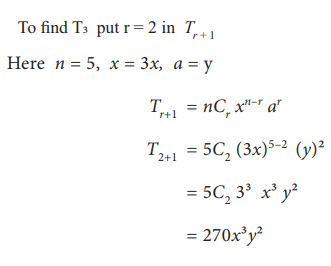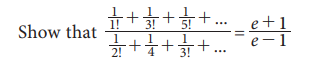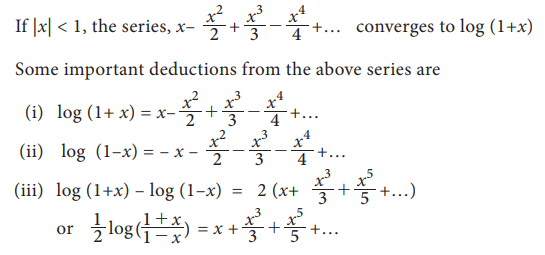Home | | Statistics 11th std | Introduction to Binomial, Exponential and Logarithmic series

# Introduction to Binomial, Exponential and Logarithmic series

Binomial, Exponential and Logarithmic series

Introduction to Binomial, Exponential and Logarithmic series

## Binomial series

A binomial is an algebraic expression of two terms. Now let us see the following binomial expansion and the number pattern we get adjacent to it.From the above, we observe that the binomial coefficients form a number pattern which is in a triangular form. This pattern is known as Pascal’s triangle. [ In pascal’s triangle, the binomial coefficients appear as each entry is the sum of the two above it.]

### Binomial theorem for a positive integral index:

For any natural number nIn the above binomial expansion, we observe,

(i)  The (r+1)th  term is denoted by T r+1  = ncr xnrar

(ii) The degree of ‘x’ in each term decreases while that of ‘a’ increases such that the sum of the power in each term equal to n

(iii) nc0, nc1, nc2, … ncr, … ncn are binomial coefficients they are also written as c0, c1, c2 … , cn

(iv) From the relation ncr = ncnr we see that the coefficients of term equidistant from the beginning and the end are equal.

### Example 7.15

Expand (2x+y)5  using binomial theorem.

### Solution:### Example 7.16

Find the middle terms of expansion (3x+y)5

### Solution:

In the expansion of (3x+y)5 we have totally 6 terms. From this the middle terms are T3 and T4Similarly we can find by putting r = 3 in Tr+1  to get T4 , then T4 =90x2y3

### Binomial theorem for a rational index:

For any rational number other than positive integer### Some important expansions:### Special cases of infinite series:### Example 7.17

Find the approximate value of 3√1002  (correct to 3 decimal places) using Binomial series.

### Solution:## Exponential series### Example 7.18### Solution:## Logarithmic series:Study Material, Lecturing Notes, Assignment, Reference, Wiki description explanation, brief detail
11th Statistics : Chapter 7 : Mathematical Methods : Introduction to Binomial, Exponential and Logarithmic series |